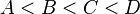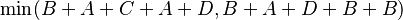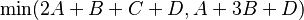# Bridge and torch problem facts for kids

Kids Encyclopedia Facts

The bridge and torch problem (also known as The Midnight Train and Dangerous crossing) is a logic puzzle that deals with four people, a bridge and a torch. It is in the category of river crossing puzzles, where a number of objects must move across a river, with some constraints.

## Story

Four people come to a river in the night. There is a narrow bridge, but it can only hold two people at a time. They have one torch and, because it's night, the torch has to be used when crossing the bridge. Person A can cross the bridge in 1 minute, B in 2 minutes, C in 5 minutes, and D in 8 minutes. When two people cross the bridge together, they must move at the slower person's pace. The question is, can they all get across the bridge if the torch lasts only 15 minutes?

## Solution

An obvious first idea is that the cost of returning the torch to the people waiting to cross is an unavoidable expense which should be minimized. This strategy makes A the torch bearer, shuttling each person across the bridge:

Elapsed Time Starting Side Action Ending Side
0 minutes A B C D
2 minutes       C D A and B cross forward, taking 2 minutes A B
3 minutes A    C D A returns, taking 1 minute    B
8 minutes          D A and C cross forward, taking 5 minutes A B C
9 minutes A       D A returns, taking 1 minute    B C
17 minutes A and D cross forward, taking 8 minutes A B C D

This strategy does not permit a crossing in 15 minutes. To find the correct solution, one must realize that forcing the two slowest people to cross individually wastes time which can be saved if they both cross together:

Elapsed Time Starting Side Action Ending Side
0 minutes A B C D
2 minutes       C D A and B cross forward, taking 2 minutes A B
3 minutes A    C D A returns, taking 1 minute    B
11 minutes A C and D cross forward, taking 8 minutes    B C D
13 minutes A B B returns, taking 2 minutes       C D
15 minutes A and B cross forward, taking 2 minutes A B C D

A second equivalent solution swaps the return trips. Basically, the two fastest people cross together on the 1st and 5th trips, the two slowest people cross together on the 3rd trip, and EITHER of the fastest people returns on the 2nd trip, and the other fastest person returns on the 4th trip.

Thus the minimum time for four people is given by the following mathematical equations: When$A < B < C < D$,$\min(B + A + C + A + D, B + A + D + B + B)$$\min(2A + B + C + D,A + 3B + D)$

## A semi-formal approach

Assume that a solution minimizes the total number of crossings. This gives a total of five crossings - three pair crossings and two solo-crossings. Also, assume we always choose the fastest for the solo-cross. First, we show that if the two slowest persons (C and D) cross separately, they accumulate a total crossing time of 15. This is done by taking persons A, C, & D: C+A+D+A = 5+1+8+1=15. (Here we use A because we know that using A to cross both C and D separately is the most efficient.) But, the time has elapsed and person A and B are still on the starting side of the bridge and must cross. So it is not possible for the two slowest (C & D) to cross separately. Second, we show that in order for C and D to cross together that they need to cross on the second pair-cross: i.e. not C or D, so A and B, must cross together first. Remember our assumption at the beginning states that we should minimize crossings and so we have five crossings - 3 pair-crossings and 2 single crossings. Assume that C and D cross first. But then C or D must cross back to bring the torch to the other side, and so whoever solo-crossed must cross again. Hence, they will cross separately. Also, it is impossible for them to cross together last, since this implies that one of them must have crossed previously, otherwise there would be three persons total on the start side. So, since there are only three choices for the pair-crossings and C and D cannot cross first or last, they must cross together on the second, or middle, pair-crossing. Putting all this together, A and B must cross first, since we know C and D cannot and we are minimizing crossings. Then, A must cross next, since we assume we should choose the fastest to make the solo-cross. Then we are at the second, or middle, pair-crossing so C and D must go. Then we choose to send the fastest back, which is B. A and B are now on the start side and must cross for the last pair-crossing. This gives us, B+A+D+B+B = 2+1+8+2+2 = 15.Bridge and torch problem Facts for Kids. Kiddle Encyclopedia.